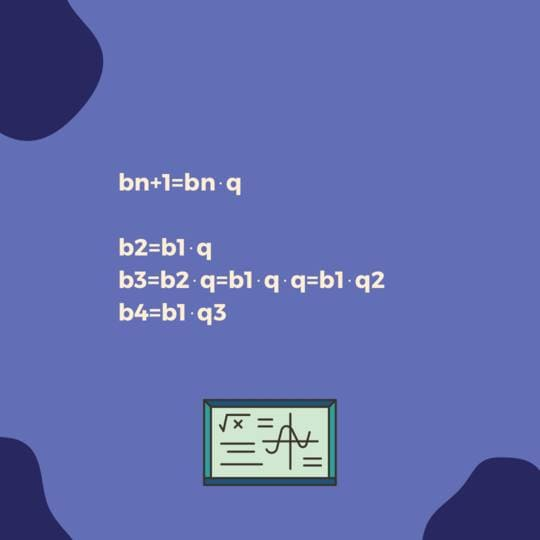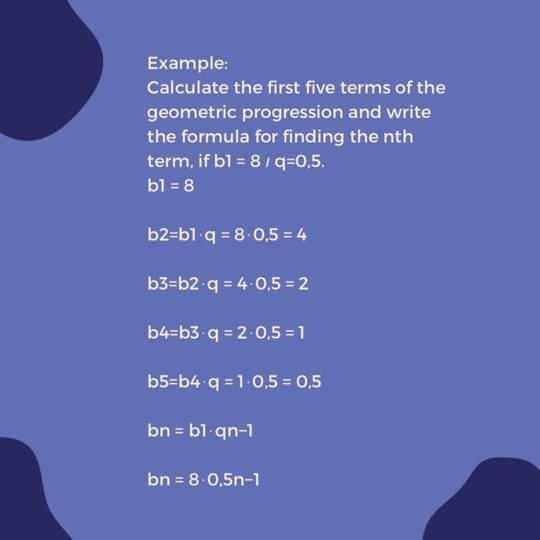# Geometric Progression

## What is a geometric progression?

Geometric progression, also known as geometric sequence, is a mathematical concept and plays a key role in various areas of human life. Geometric progression is used in various fields of study, such as finance, geometry, computer science, and telecommunications, and is essential for understanding many fundamental principles and applications in these areas. You have heard many times in maths classes that maths is an important subject in our lives. In this article we will look at what a geometric progression is, how it is used in life and why it is so important in mathematics.

For example:### The role of geometric progression in finance

Geometric progression is the basis for understanding many financial and economic principles. For example, geometric progression is used to calculate interest on loans or deposits. If you invest \$1,000 at 5% per annum for 5 years, your deposit will grow to \$1,276 after 5 years. This is because interest accrues not only on the original deposit amount, but also on the interest already accrued. This is an example of using progression in financial calculations.

## Geometric Progression Properties in geometry

Progression also has applications in geometry. In progression with a denominator of 2, each successive member is twice as large. If we construct squares with corresponding sides, then we get a spiral of squares, which is this very progression. This spiral is named Fibonacci.

It also plays a major role in technical sciences, including computer science, telecommunications. In telecommunications, it is often used when transmitting some kind of data. Signals are transmitted at increasing speeds, starting at lower speeds and then gradually increasing the data rate.

For example:### What is the difference between geometric and arithmetic progression?

Arithmetic is a mathematical concept denoting a number sequence in which every successive element differs from the last by a permanent value, called a difference. This distinguishes it from a geometrical series, where every subsequent item is obtained by multipleting the prior item with a permanent denominator.

In a simple series, every item in the series changes by the way that the previous item does, allowing each item to either increase or decrease at a constant rate. In contrast, in a geometrical series, every element can either increase or decrease at a constant denominator. These properties of progressions lead to different uses in mathematics and in real-world scenarios.

All progressions have distinct characteristics that set them apart from one another. The key point of distinction in an arithmetical progression, every element can increase or decrease by a constant amount, whereas in a geometric progression every element can either multiply or divide to a constant denominator. This means that an arithmetic progression has a constant rate of change, whereas a Geometric series has a permanent change rate. These differences in the behaviour of the the two types of progresse lead to different uses and features in mathematics and in real-world situations.

## Geometric Progression Calculator

Online calcs provide easy-to-access tools to calculate various parameters easily. These calculators can be found on many mathematics websites and could save users time by eliminating the need for manual calculations.

A key feature of the geometric series calcs is its ability to summarise the amount of the start n term of a series. Having entered the starting term, the total coefficient and the total number of terms to be summed, users can quickly obtain a solution without the have to calculate manually. In addition, the calculator can determine other important parameters. By entering known parameters, the calculator can automatically solve all missing values.

It is crucial to bear in mind that a geometric progression calculator is merely a tool that can aid in completing tasks, but cannot serve as a substitute for comprehending the theory and correctly solving problems. Therefore, it is highly recommended to double-check the accuracy of the data entered and acquaint oneself with the fundamental principles of solving problems of this kind before utilizing a geometric progression calculator.

## Role of geometric progression in our life

The significance of geometric progression in our lives cannot be overstated. We employ it when performing calculations on a calculator or devising a budget. For instance, if we aim to set aside a specific sum of money every month, we can utilize this progression to determine the required payments.

Moreover, it plays a significant role in our comprehension of ourselves and the world around us. In nature, we frequently observe instances of geometric progression, such as the rise in animal populations or tree growth. Our bodies can also be described by geometric progression, like the growth of hair or nails. For those who wish to delve deeper into this subject, the TeMa platform's Maths Tutor can be used. We wish you all the best in your mathematical pursuits!